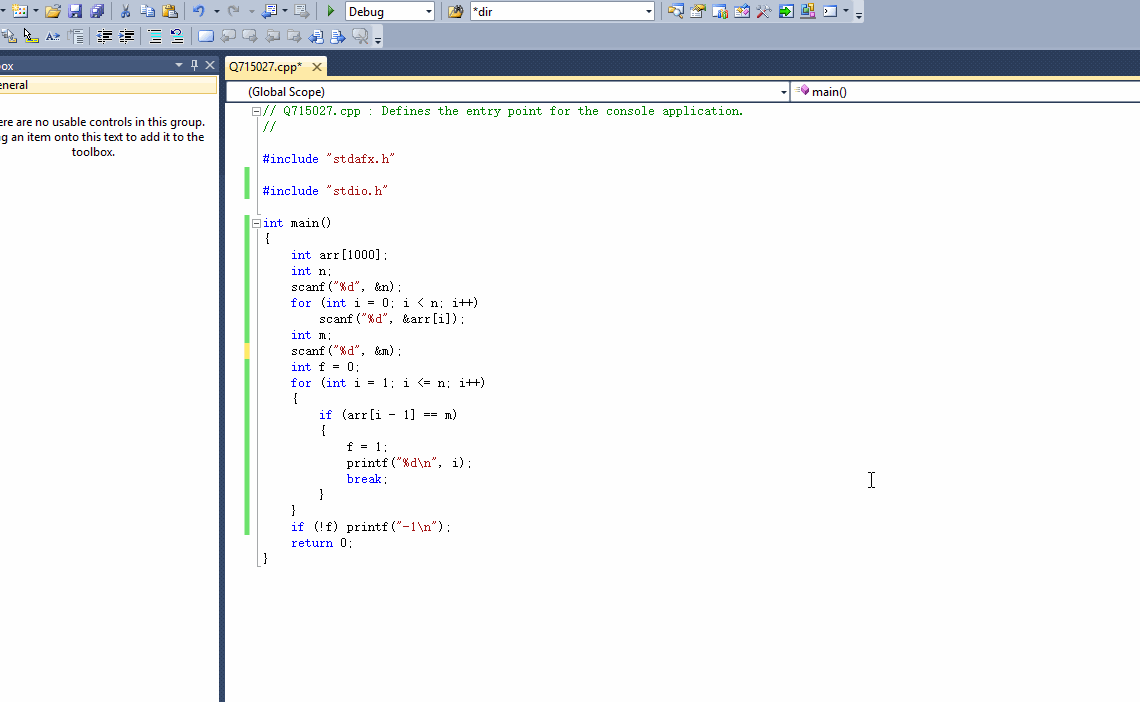6
1 9 4 8 3 9
9

2

1 <= n <= 1000。

1个回答

# 如果问题得到解决，请点我回答左上角的采纳和向上的箭头，谢谢

``````#include "stdio.h"

int main()
{
int arr;
int n;
scanf("%d", &n);
for (int i = 0; i < n; i++)
scanf("%d", &arr[i]);
int m;
scanf("%d", &m);
int f = 0;
for (int i = 1; i <= n; i++)
{
if (arr[i - 1] == m)
{
f = 1;
printf("%d\n", i);
break;
}
}
if (!f) printf("-1\n");
return 0;
}
``````